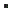What is LowValue and HighValue?"LowValue" (and "HighValue") are alias names for the lowest (highest) possible value for a certain type. When using a SeekBy method, you will sometimes need to provide a correct LowValue or HighValue for a parameter if you do not have a specific value for it, since all parameters are required.C++ ```const VARIANT_BOOL LowValue_Bool = VARIANT_TRUE; const VARIANT_BOOL HighValue_Bool = VARIANT_FALSE; const BYTE LowValue_Byte = 0; const BYTE HighValue_Byte = BYTE_MAX; const short LowValue_Short = SHORT_MIN; // See (remark) below const short LowValue_UShort = 0; // See (remark) below const short HighValue_Short = SHORT_MAX; const long LowValue_Long = LONG_MIN; // See (remark) below const long LowValue_ULong = 0; // See (remark) below const long HighValue_Long = LONG_MAX; const double LowValue_Double = -999999999999999.0; const double HighValue_Double = 999999999999999.0; //const enum LowValue_Enum = lowest specific value for that enum (see Enum List) //const enum HighValue_Enum = lowest specific value for that enum (see Enum List) const COleVariant LowValue_String = ""; const COleVariant HighValue_String = CString('\xff', 255); const DATE LowValue_Date = COleDateTime().m_dt; const DATE HighValue_Date = COleDateTime(9999,12,31,0,0,0).m_dt; ```C# ```const bool LowValue_Bool = true; const bool HighValue_Bool = false; const byte LowValue_Byte = 0; const byte HighValue_Byte = 255; const short LowValue_Short = -32768; // See (remark) below const short LowValue_UShort = 0; // See (remark) below const short HighValue_Short = 32767; const long LowValue_Long = -2147483648; // See (remark) below const long LowValue_ULong = 0; // See (remark) below const long HighValue_Long = 2147483647; const double LowValue_Double = -999999999999999.0; const double HighValue_Double = 999999999999999.0; //const enum LowValue_Enum = lowest specific value for that enum (see Enum List) //const enum HighValue_Enum = lowest specific value for that enum (see Enum List) const string LowValue_String = ""; const string HighValue_String = new string('\xff', 255); const DateTime LowValue_Date = new DateTime(); const DateTime HighValue_Date = new DateTime(9999,12,31); ```VBS ```Const LowValue_Bool = True Const HighValue_Bool = False Const LowValue_Byte = 0 Const HighValue_Byte = 255 Const LowValue_Short = -32768 ' See (remark) below Const LowValue_UShort = 0 ' See (remark) below Const HighValue_Short = 32767 Const LowValue_Long = -2147483648 ' See (remark) below Const LowValue_ULong = 0 ' See (remark) below Const HighValue_Long = 2147483647 Const LowValue_Double = -999999999999999.0 Const HighValue_Double = 999999999999999.0 'Const LowValue_Enum = lowest specific value for that enum (see Enum List) 'Const HighValue_Enum = lowest specific value for that enum (see Enum List) Const LowValue_String = "" HighValue_String = String(255, Chr(255)) Dim LowValue_Date Const HighValue_Date = #12/31/9999# ```VB.NET ```Const LowValue_Bool As Boolean = True Const HighValue_Bool As Boolean = False Const LowValue_Byte As Byte = 0 Const HighValue_Byte As Byte = 255 Const LowValue_Short As Short = -32768 ' See (remark) below Const LowValue_UShort As Short = 0 ' See (remark) below Const HighValue_Short As Short = 32767 Const LowValue_Long As Long = -2147483648.0# ' See (remark) below Const LowValue_ULong As Long = 0 ' See (remark) below Const HighValue_Long As Long = 2147483647 Const LowValue_Double As Double = -999999999999999.0# Const HighValue_Double As Double = 999999999999999.0# 'Const LowValue_Enum = lowest specific value for that enum (see Enum List) 'Const HighValue_Enum = lowest specific value for that enum (see Enum List) Const LowValue_String As String = "" ReadOnly HighValue_String As String = New String(Chr(255), 255) ReadOnly LowValue_Date As Date = New Date() Const HighValue_Date = #12/31/9999# ```

RemarkTo allow compatibility with VBScript, the Venice SDK only (with a few exceptions) uses types which are supported in VBScript. For the SeekBy methods, this means that while there are key segments that are technically of type "unsigned short" (16bit unsigned) or "unsigned long" (32bit unsigned) these are set to signed types short and long. For the LowValue however, this means there are 2 potential "lowest" values for that key segment. You will have to match the correct signed or unsigned version of the LowValue or the SeekBy method may not behave as expected.